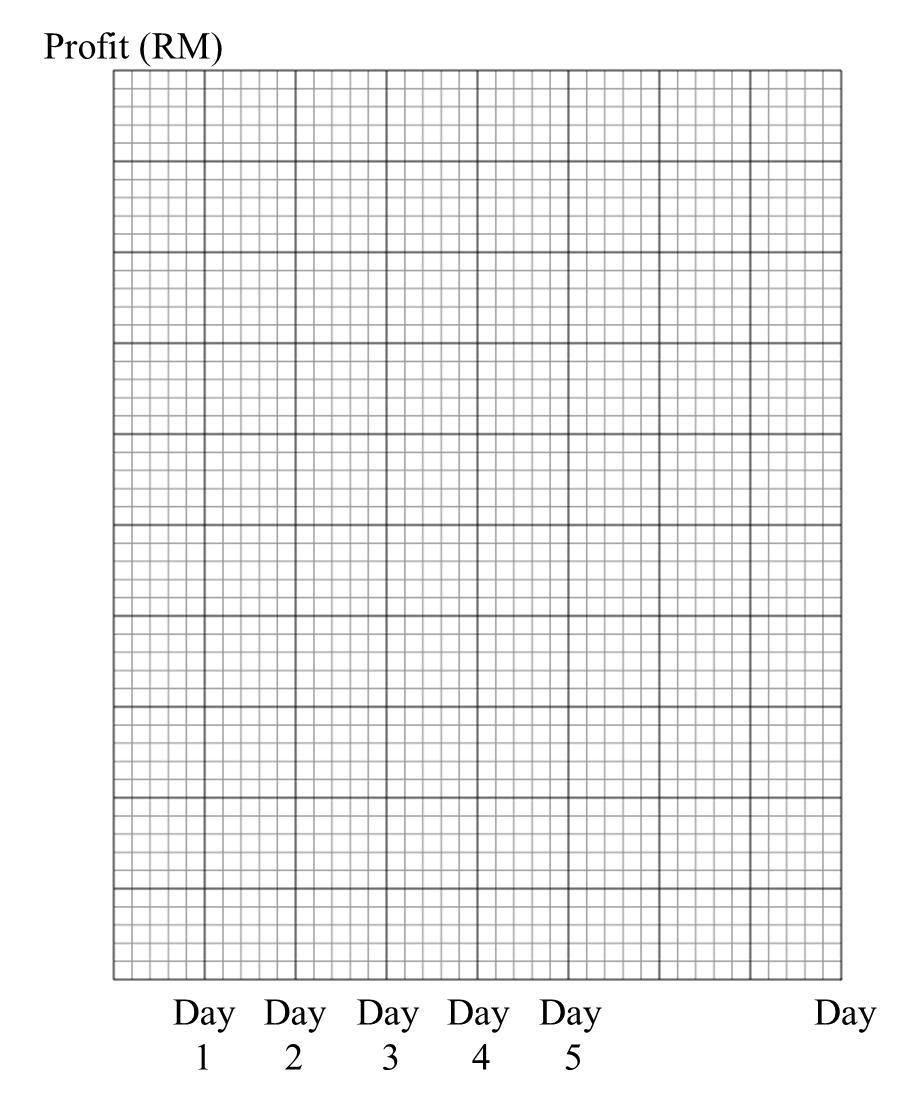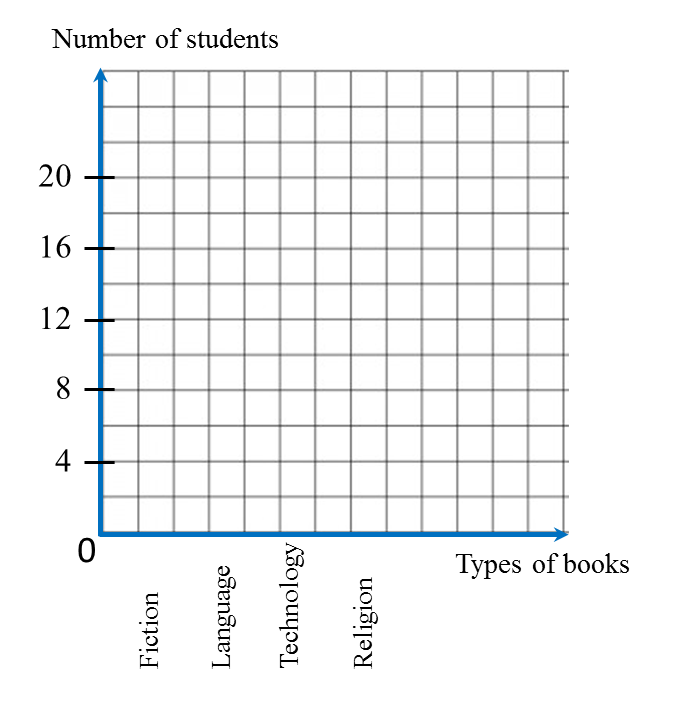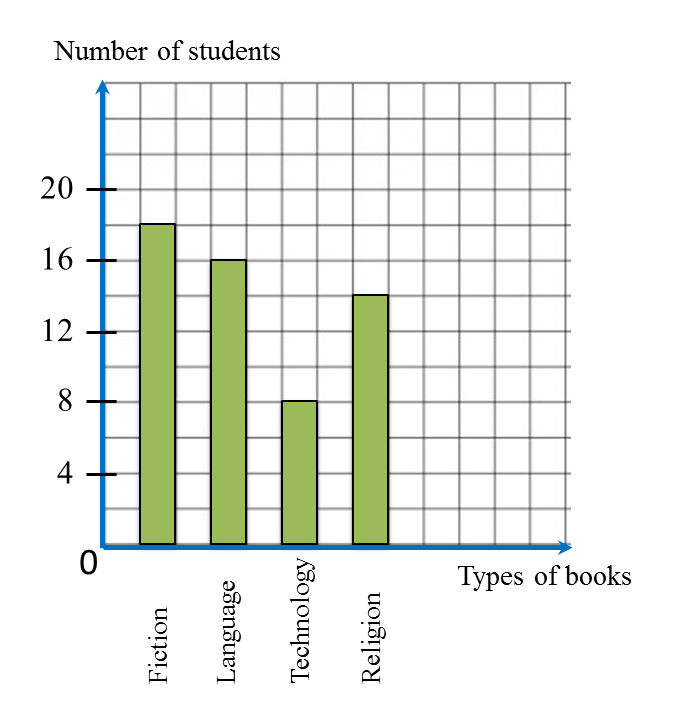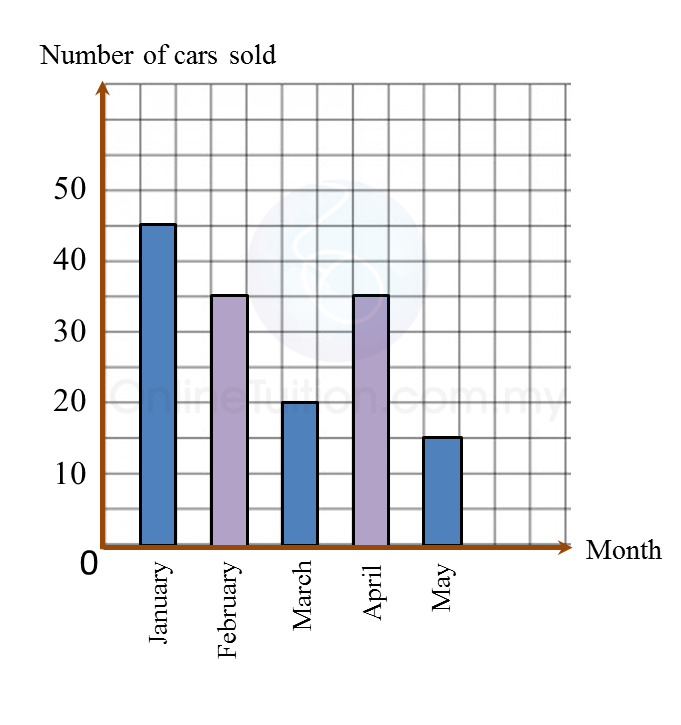13.2.2 Statistics (I), PT3 Focus Practice

13.2.2 Statistics (I), PT3 Focus Practice

Question 4:
Table below shows the profit made from the sales of coconut at a stall over five days.

 Day Day 1 Day 2 Day 3 Day 4 Day 5 Profit (RM) 280 200 200 360 320
On diagram in the answer space, draw a line graph to represent all the information in the Table. Use the scale 2 cm to RM80 on the vertical axis.

:Solution:Question 5:
Table below shows the number of students who read four types of books.

 Types of books Fiction Language Technology Religion Number of students 18 16 8 14
On diagram in the answer space, draw a bar chart to represent all the information in the Table.

:Solution:Question 6:
Diagram below is an incomplete bar chart which shows the number of cars sold in a shop for duration of five months.(a) The number of cars sold for the five months is 150 units. The number of cars sold in February is equal to the number of cars sold in April.
Complete the bar chart in the Diagram.
(b) Find the percentage of cars sold in January.

Solution:
(a)(b)